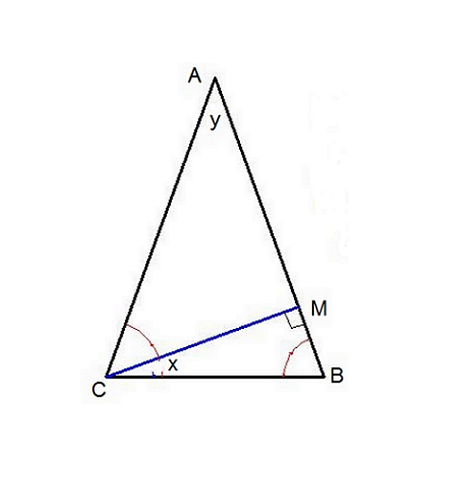# A geometry problem by Ahmed Moh AbuBakr

Geometry Level 2$ABC$ is an isosceles triangle such that $AB = AC$ and $\frac {\angle BAC}{\angle MCB} = \frac a b$ for coprime positive integers $a,b$. What is the value of $a+b$?

×